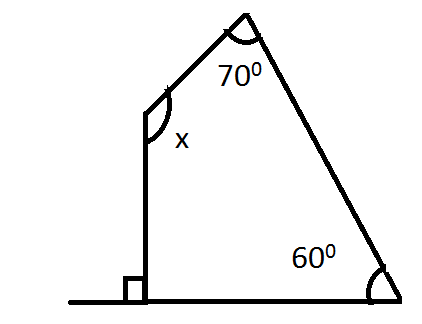QuestionAnswers

# Find the angle measure x in the following figure?Verified
129.9k+ views
Hint: The Quadrilateral or quadrangle is a shape with four sides. to be considered as a quadrangle. the shape must
1) Have four straight sides
2) Be a flat shape (2dimentional)
3) Be a closed figure.
4) Edges and vertices $= {\text{ }}4$
The word quadrilateral is derived from the Latin word quadric, a variant of four sides and the latus meaning side quadrilateral are either convex or concave.
The interior angle of a simple quadrilateral ABCD adds up to ${360^0}\;$arc.
i.e. $\angle A + {\text{ }}\angle {\text{ }}B{\text{ }} + {\text{ }}\angle {\text{ }}C{\text{ }} + {\text{ }}\angle {\text{ }}D = {\text{ }}{360^0}$.

According to the question, there are 4 Angles in a Quadrilateral.
Let$\angle {\text{ }}A = 90,{\text{ }}\angle {\text{ }}B = {\text{ }}x,{\text{ }}\angle {\text{ }}C = {\text{ }}70,\angle {\text{ }}D = {\text{ }}60$.
Since in quadrilateral sum of interior angles is equal to 3600.
$\angle {\text{ }}A{\text{ }} + {\text{ }}\angle {\text{ }}B{\text{ }} + {\text{ }}\angle {\text{ }}C{\text{ }} + {\text{ }}\angle {\text{ }}D = {360^0}$
$90 + {\text{ }}x{\text{ }} + {\text{ }}70{\text{ }} + {\text{ }}60{\text{ }} = {360^0}$
$\;\left( {90{\text{ }} + {\text{ }}60} \right){\text{ }} + {\text{ }}x{\text{ }} = {\text{ }}360{\text{ }} - 70$
$150{\text{ }} + {\text{ }}x{\text{ }} = 290$
$x{\text{ }} = {\text{ }}290{\text{ }}-{\text{ }}150$
$x{\text{ }} = {\text{ }}140$
$\angle {\text{ }}B = 140$

Note: A quadrilateral is a shape of $4$ sides for which any quadrilateral we draw a diagonal line to divide it into two triangles. Each triangle has an angle sum of $180$degree.
Therefore, the total angle sum of a quadrilateral is$360$. A quadrilateral cannot have $3$ Obtuse angle where an obtuse angle is an angle that has a measure that is greater than${90^0}$.